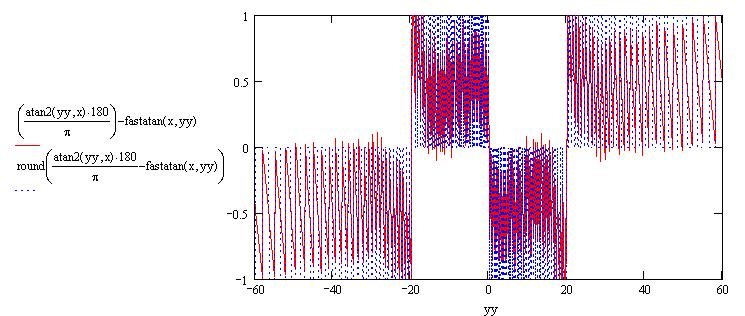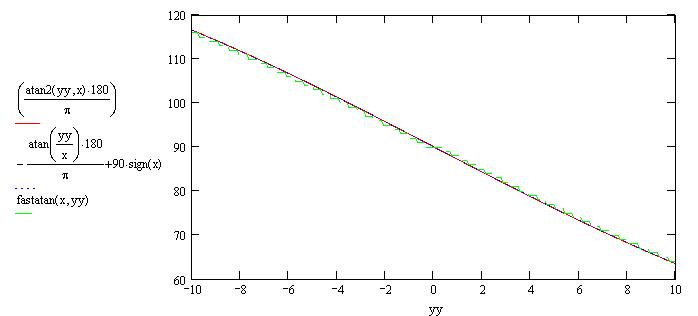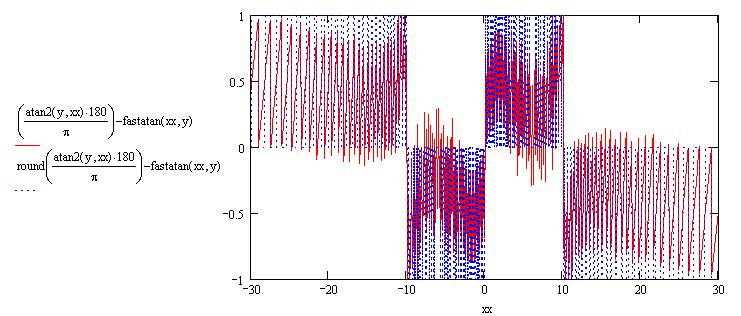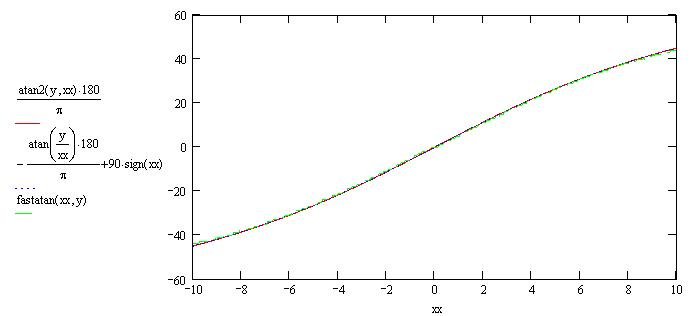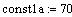this one is empirical, i started from 0.25 and moved around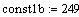This is how I am simulating
16 bit integer math with this
software suite.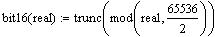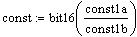change const2 to 1.0 to get result in radians (I personally prefer working in degrees)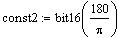this one here just means "quarter circle" so pi/4 or 90 degrees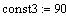main formula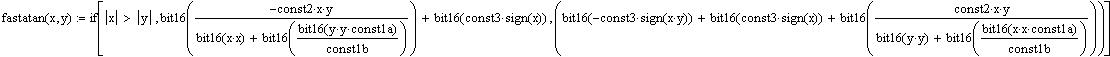the sign-related stuff can be done very quickly with some bitwise and if or case statements. Note that this time there are 2 divisions as you want to multiply by const1a before dividing by const1b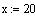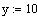tweak these to see what happens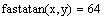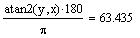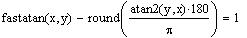comparison between atan2 and its approximation
error in degrees over range